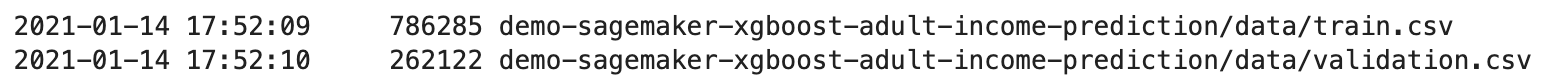Amazon Web Services 文档中描述的 Amazon Web Services 服务或功能可能因区域而异。要查看适用于中国区域的差异，请参阅 中国的 Amazon Web Services 服务入门 (PDF)

# 步骤 3：下载、浏览和转换数据集

## 使用 SHAP 加载成人人口普查数据集

``````import shap
feature_names = list(X.columns)
feature_names``````
###### 注意

``%conda install -c conda-forge shap``

``````import IPython
IPython.Application.instance().kernel.do_shutdown(True)``````

`feature_names`列表对象应返回以下功能列表：

``````['Age',
'Workclass',
'Education-Num',
'Marital Status',
'Occupation',
'Relationship',
'Race',
'Sex',
'Capital Gain',
'Capital Loss',
'Hours per week',
'Country']``````

## 数据集概述

``````display(X.describe())
hist = X.hist(bins=30, sharey=True, figsize=(20, 10))``````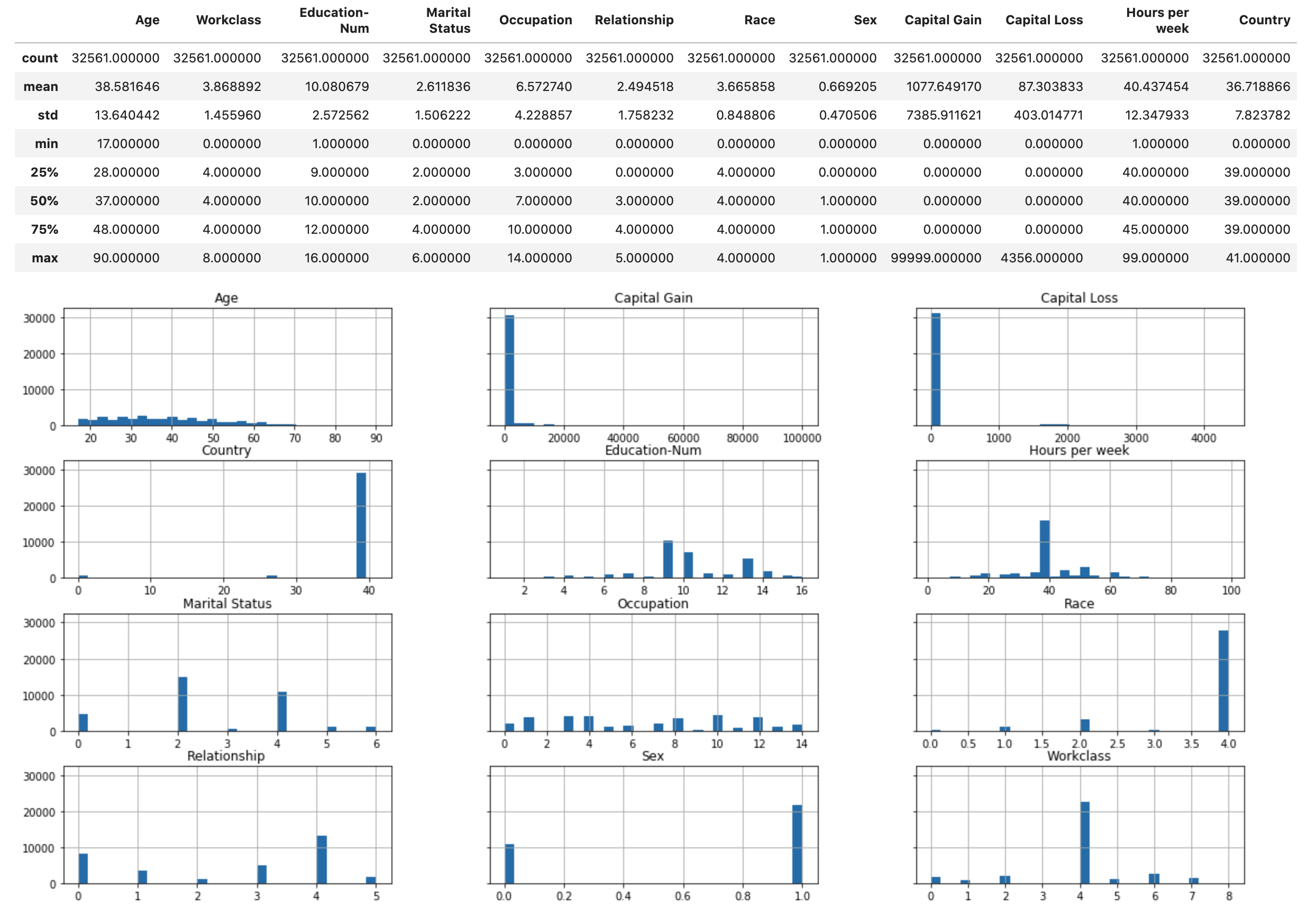## 将数据集拆分为训练、验证和测试数据集

``````from sklearn.model_selection import train_test_split
X_train, X_test, y_train, y_test = train_test_split(X, y, test_size=0.2, random_state=1)
X_train_display = X_display.loc[X_train.index]``````

``````X_train, X_val, y_train, y_val = train_test_split(X_train, y_train, test_size=0.25, random_state=1)
X_train_display = X_display.loc[X_train.index]
X_val_display = X_display.loc[X_val.index]``````

``````import pandas as pd
train = pd.concat([pd.Series(y_train, index=X_train.index,
name='Income>50K', dtype=int), X_train], axis=1)
validation = pd.concat([pd.Series(y_val, index=X_val.index,
name='Income>50K', dtype=int), X_val], axis=1)
test = pd.concat([pd.Series(y_test, index=X_test.index,
name='Income>50K', dtype=int), X_test], axis=1)``````

``train``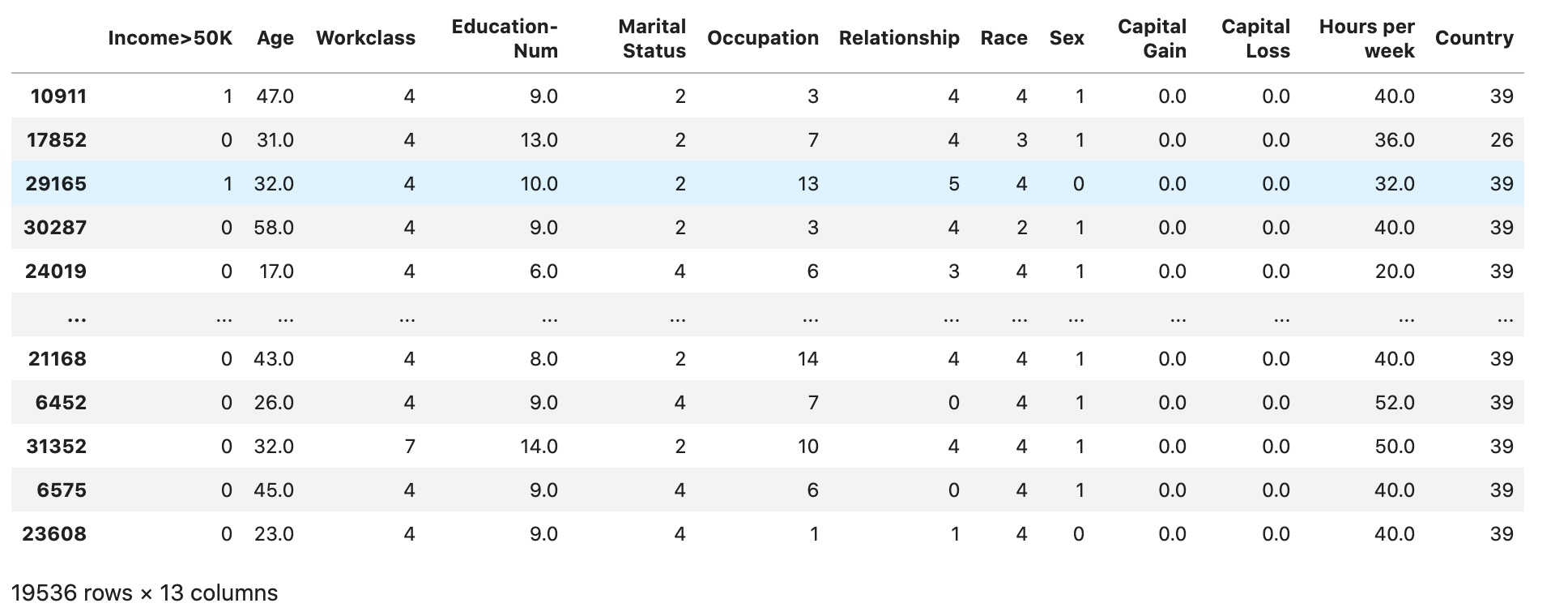``validation``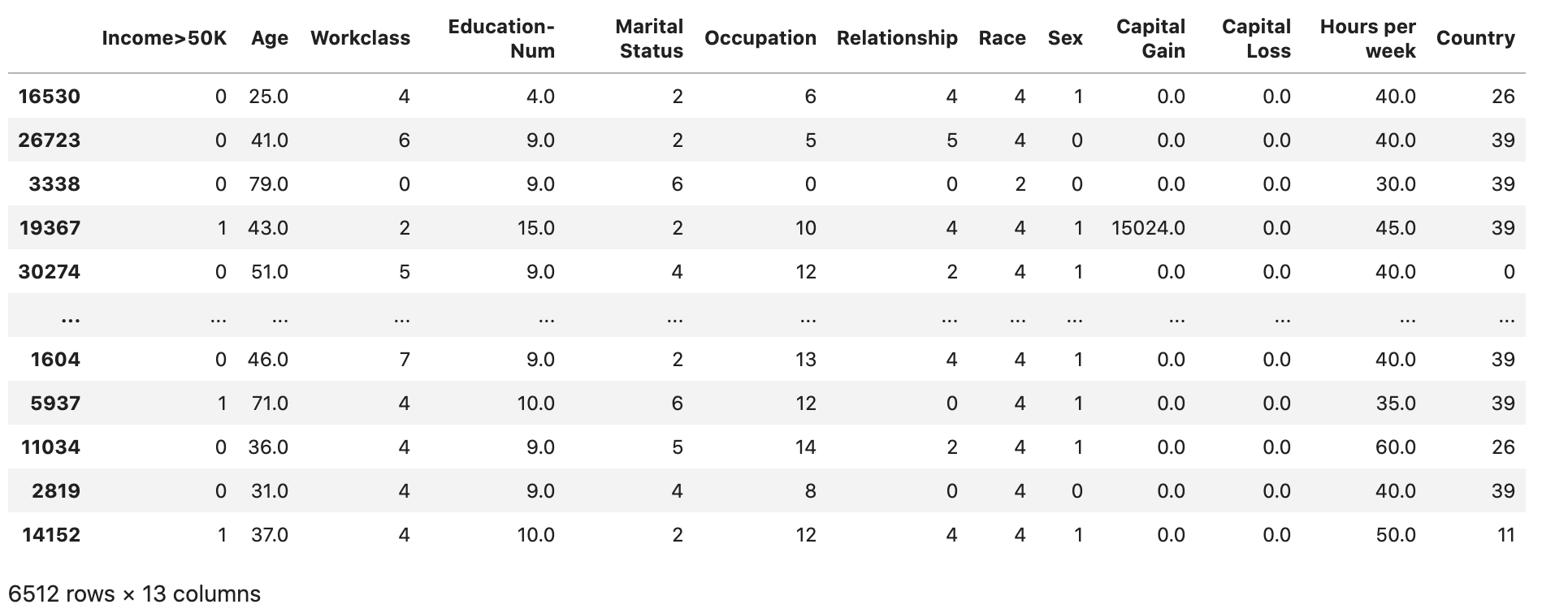``test``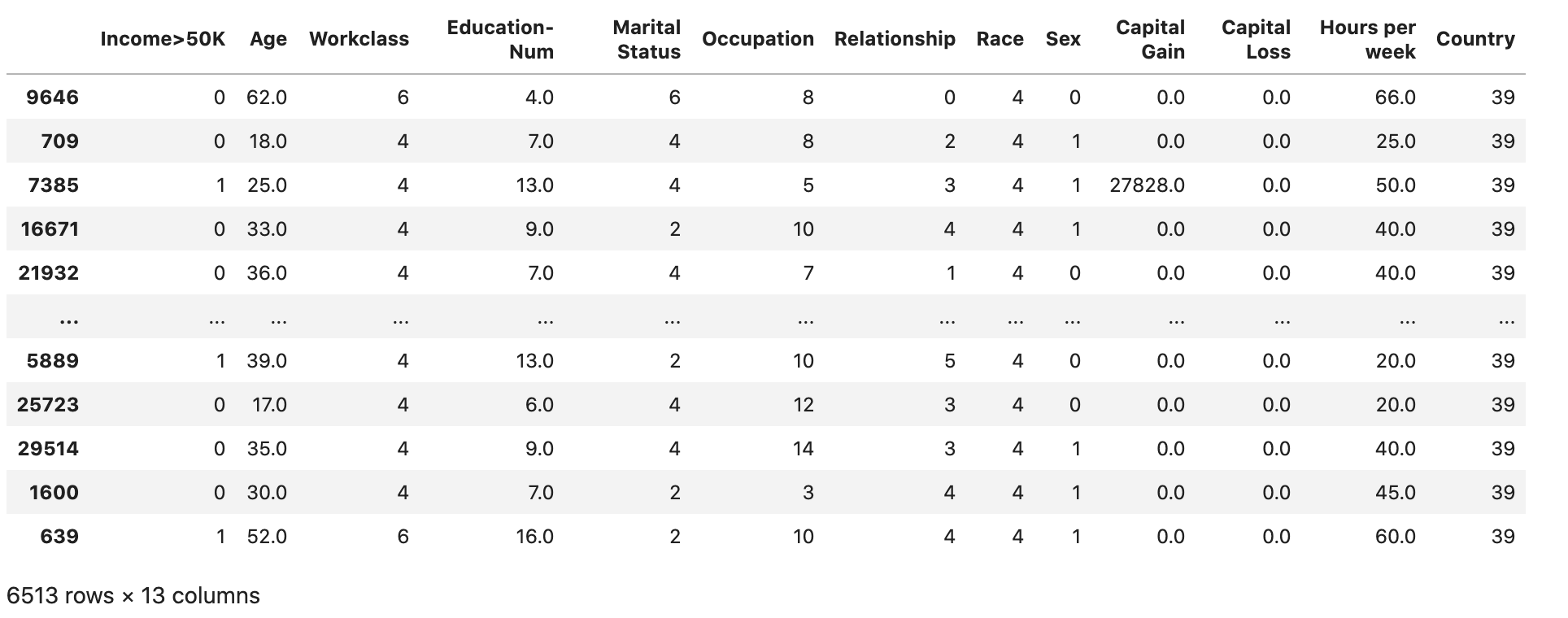## 将训练和验证数据集转换为 CSV 文件

`train``validation`数据框对象转换为 CSV 文件，以匹配 XGBoost 算法的输入文件格式。

``````# Use 'csv' format to store the data
# The first column is expected to be the output column

## 将数据集上传到 Amazon S3

``````import sagemaker, boto3, os
bucket = sagemaker.Session().default_bucket()
``! aws s3 ls {bucket}/{prefix}/data --recursive``